Algebra 1 8-1 Complete Lesson: Adding and Subtracting Polynomials
starstarstarstarstarstarstarstarstarstar
4.5 (1 rating)
by Matthew Richardson
| 29 Questions
Note from the author:
A complete formative lesson with embedded slideshow, mini lecture screencasts, checks for understanding, practice items, mixed review, and reflection. I create these assignments to supplement each lesson of Pearson's Common Core Edition Algebra 1, Algebra 2, and Geometry courses. See also mathquest.net and twitter.com/mathquestEDU.The outlined content above was added from outside of Formative.1
2
3
1
10 pts
Solve It! The costs of admission and parking for a museum and an aquarium are given. What is the total cost of m people in n cars visiting only the museum?
5m + 15n
20m
15m + 5n
5m + 5n
2
10 pts
Solve It! What is the total cost of m people in n cars visiting only the aquarium?
20m + 6n
26m
6m + 20n
26n
3
10 pts
Solve It! What is the total cost of m people in n cars visiting BOTH the museum and aquarium?
11m + 36n
51m
51n
35m + 11n4
4
10 pts
Problem 1 Got It?
A
B
C
D5
5
10 pts
Problem 1 Got It?
A
B
C
D6
6
10 pts
Problem 1 Got It?
A
B
C
D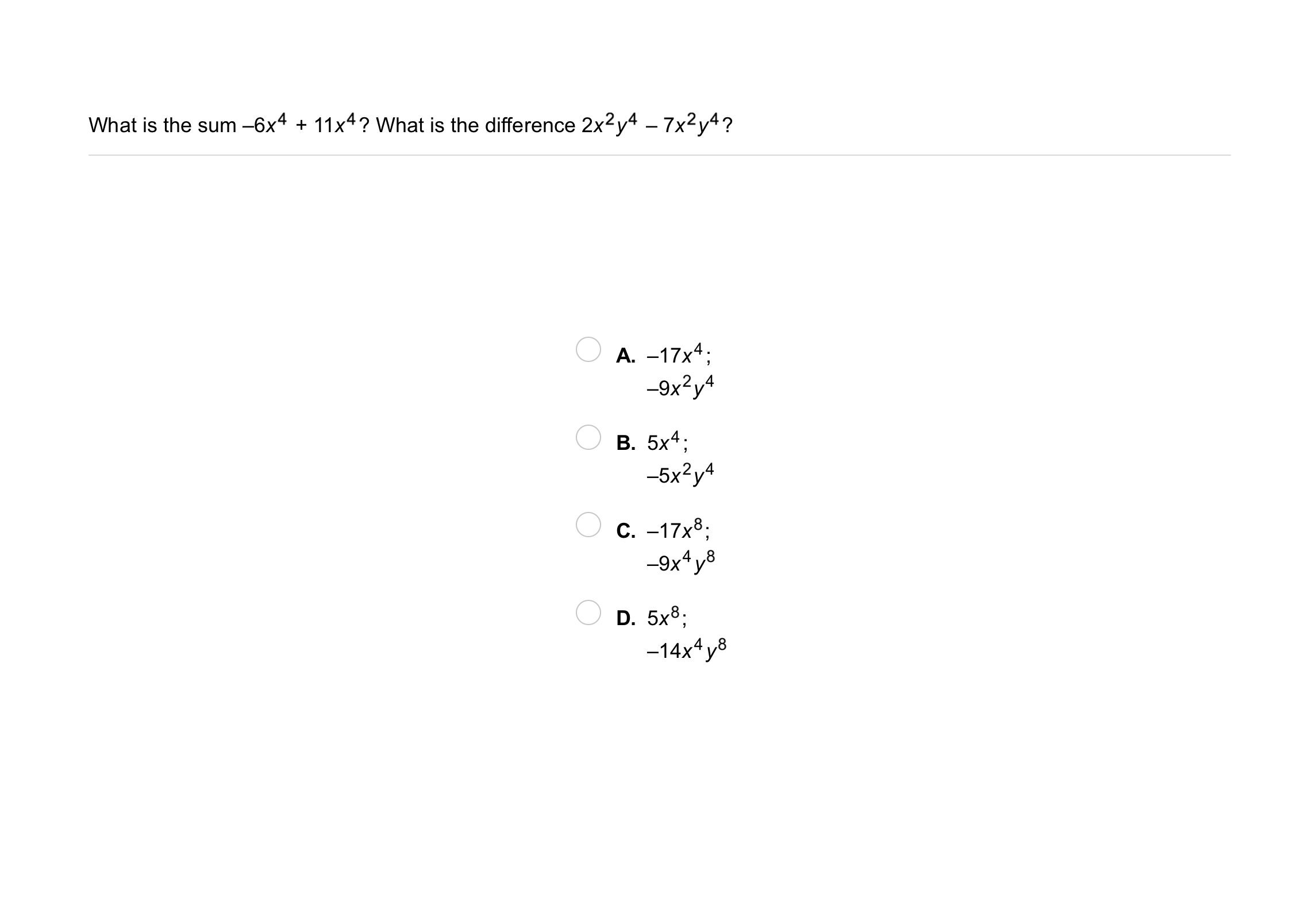7
7
10 pts
Problem 2 Got It?
A
B
C
D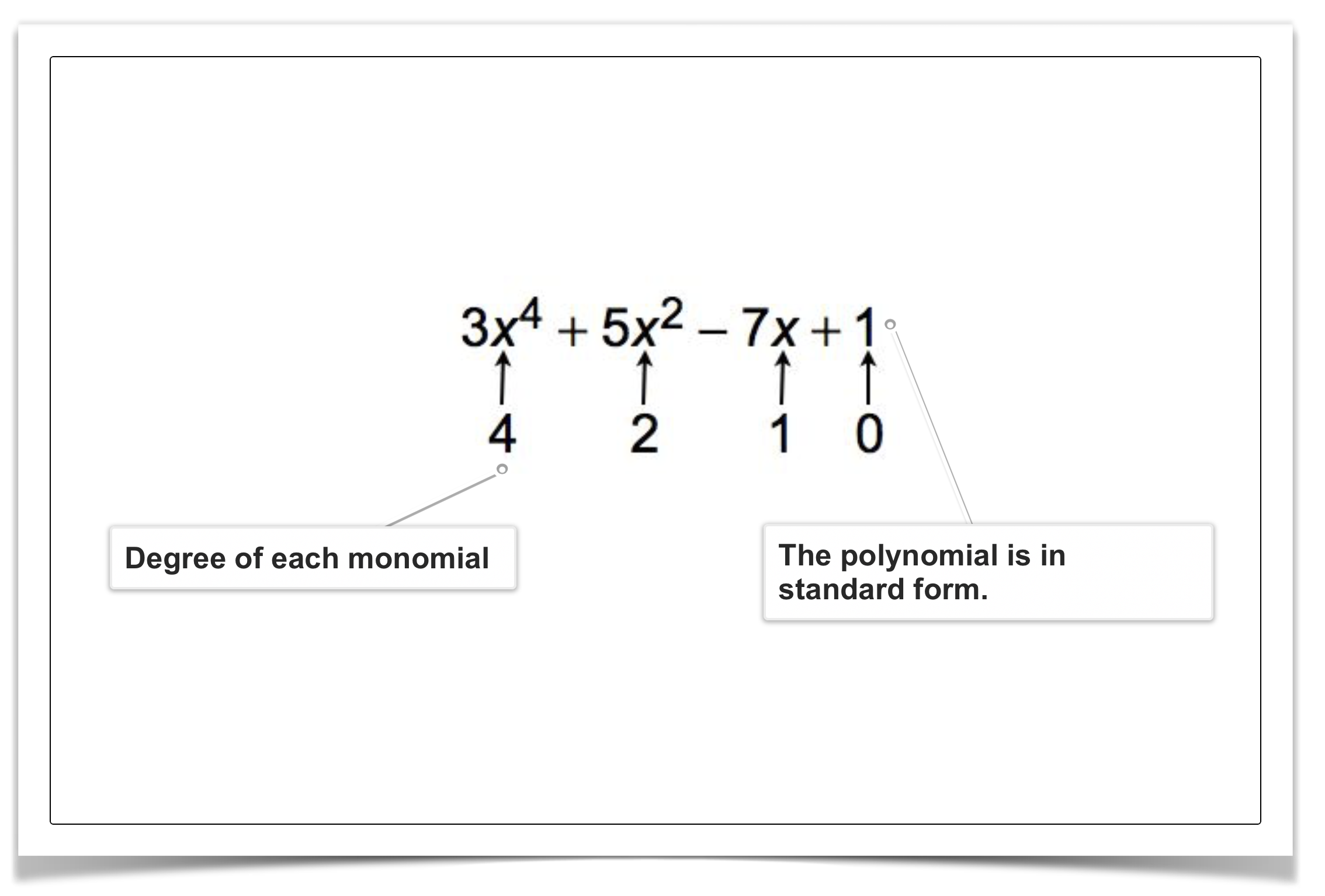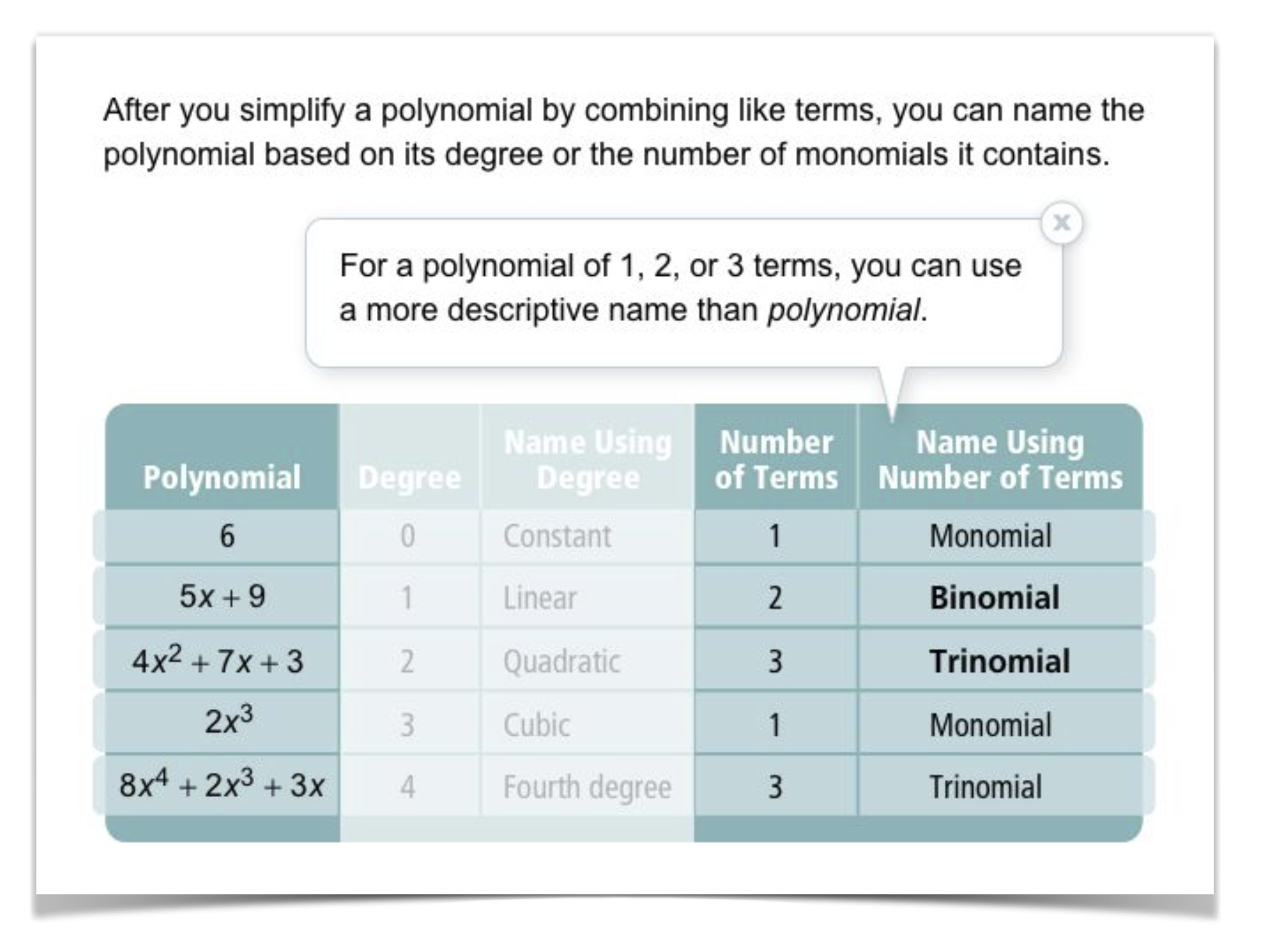8
10 pts
Problem 3 Got It? Write the expression in standard form.

9
10 pts
Problem 3 Got It? What is the name of the polynomial from the previous item based on its degree and number of terms.
• linear
• cubic
• binom
• trinomial
• Polynomial name based on degree
• Polynomial name based on number of terms
10
10 pts
Problem 3 Got It? Reasoning: How does writing a polynomial in standard form help you name the polynomial?11
11
10 pts
Problem 4 Got It?
A
B
C
D12
12
10 pts
Problem 5 Got It?
A
B
C
D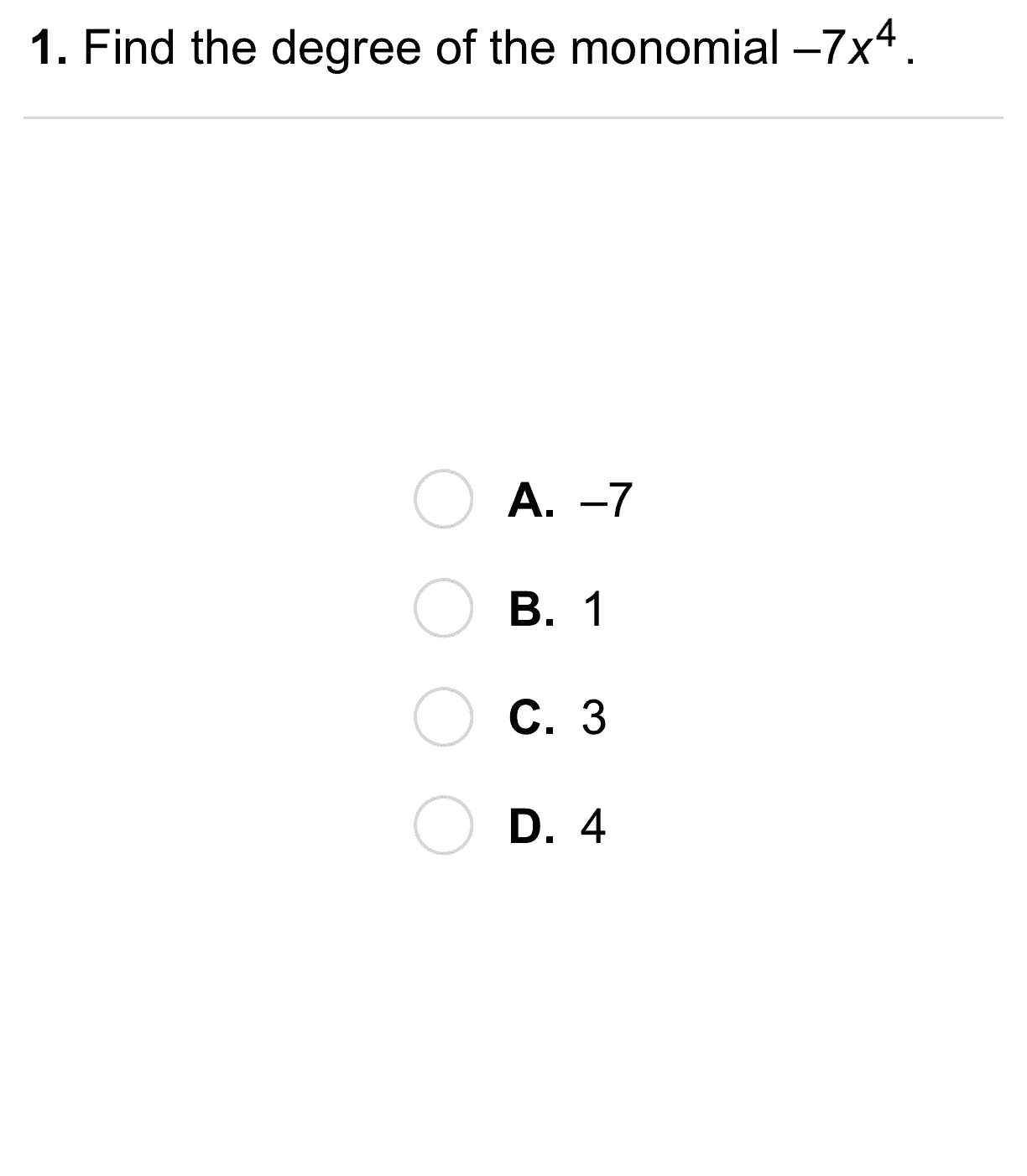13
13
10 pts
A
B
C
D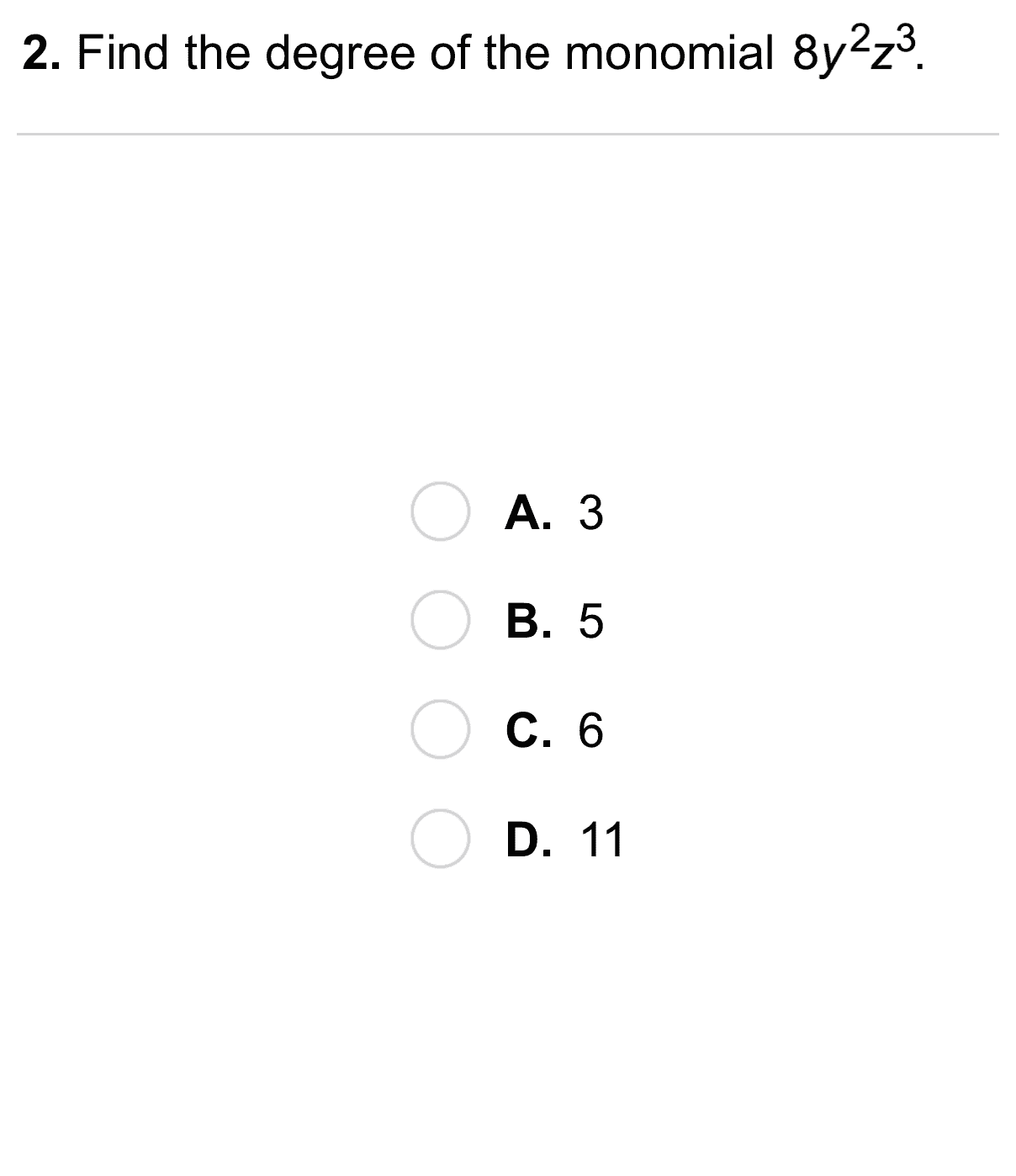14
14
10 pts
Find the degree of the monomial.

A
B
C
D15
15
10 pts
A
B
C
D16
16
10 pts
A
B
C
DClassifying Polynomials17
10 pts
Vocabulary: Classify the polynomial based on its degree and number of terms.
constant monomial
cubic monomial
18
10 pts
Vocabulary: Classify the polynomial based on its degree and number of terms.
Your response should contain two words and one space. See the previous item for formatting.20
10 pts
Review Lesson 7-7: Match each exponential function with its growth factor.

• Growth factor: 2
• Growth factor: 3
• Growth factor: 4
21
10 pts
Review Lesson 7-7: Match each exponential function with its initial amount.

• Initial amount: 2
• Initial amount: 3
• Initial amount: 7
22
10 pts
Review Lesson 5-1: Find the slope of the line that passes each pair of points.
• Slope: -2/3
• Slope: -2/5
• Slope: -8/3
• Slope: 8
• (0, 2), (5, 0)
• (3, -7), (4, 1)
• (-2, 8), (1, 0)
• (9, -6), (0, 0)
23
10 pts
Review Lesson 7-2: Simplify the expression.

24
10 pts
Review Lesson 7-2: Simplify the expression.

25
10 pts
Review Lesson 7-2: Simplify the expression.26
10 pts
Vocabulary Review: Categorize each as an expression or equation.

• Expression
• Equation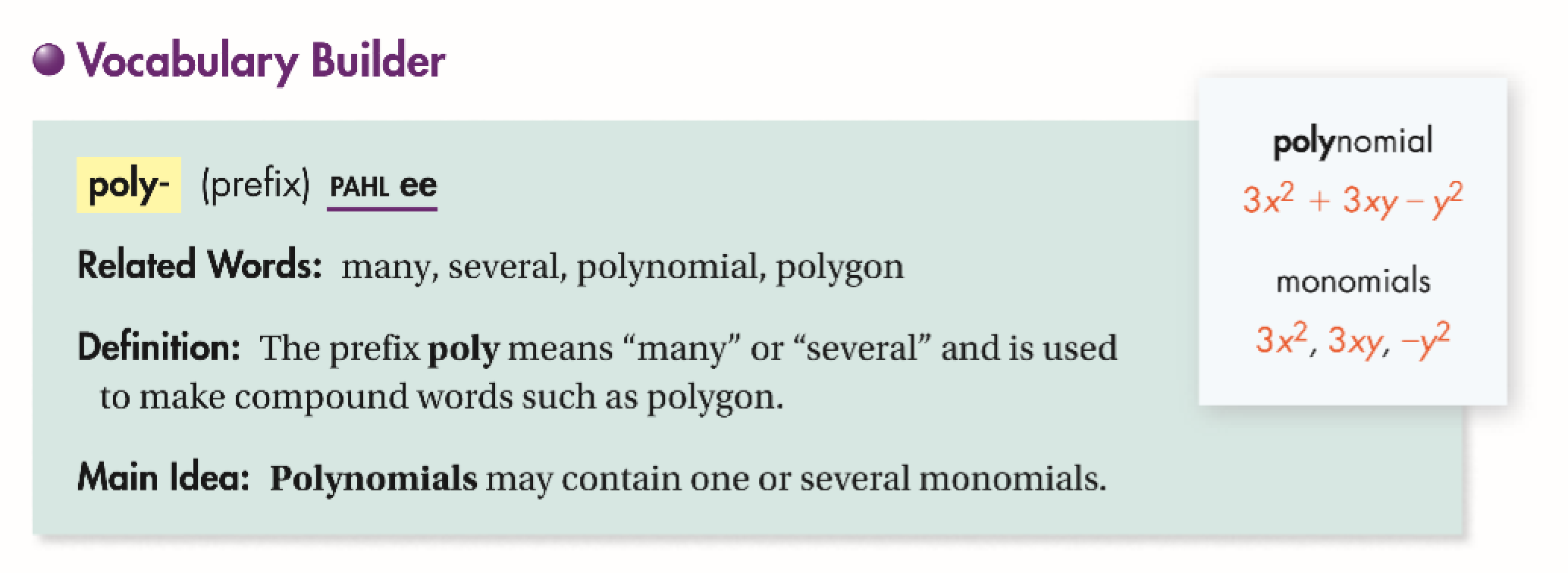27
10 pts
Use Your Vocabulary: Complete each statement with the correct word from the list. Use each word only once.
• polygon
• polygraph
• polynomial
• A monomial or a sum of monomials is a __?__.
• A __?__ test measures many tiny physical reactions of a person who is being questioned in order to determine whether that person is telling the truth.
• A triangle is one example of a __?__.
28
100 pts
Notes: Take a clear picture or screenshot of your Cornell notes for this lesson. Upload it to the canvas. Zoom and pan as needed.

For a refresher on the Cornell note-taking system, click here.
29
10 pts
Reflection: Math Success
Add to my formatives list

Formative uses cookies to allow us to better understand how the site is used. By continuing to use this site, you consent to the Terms of Service and Privacy Policy.